xEncyclopedia
In mathematics
Mathematics
Mathematics is the study of quantity, space, structure, and change. Mathematicians seek out patterns and formulate new conjectures. Mathematicians resolve the truth or falsity of conjectures by mathematical proofs, which are arguments sufficient to convince other mathematicians of their validity...

, the spectral radius of a square matrix
Matrix (mathematics)
In mathematics, a matrix is a rectangular array of numbers, symbols, or expressions. The individual items in a matrix are called its elements or entries. An example of a matrix with six elements isMatrices of the same size can be added or subtracted element by element...

or a bounded linear operator is the supremum
Supremum
In mathematics, given a subset S of a totally or partially ordered set T, the supremum of S, if it exists, is the least element of T that is greater than or equal to every element of S. Consequently, the supremum is also referred to as the least upper bound . If the supremum exists, it is unique...

among the absolute value
Absolute value
In mathematics, the absolute value |a| of a real number a is the numerical value of a without regard to its sign. So, for example, the absolute value of 3 is 3, and the absolute value of -3 is also 3...

s of the elements in its spectrum
Spectrum of a matrix
In mathematics, the spectrum of a matrix is the set of its eigenvalues. This notion can be extended to the spectrum of an operator in the infinite-dimensional case.The determinant equals the product of the eigenvalues...

, which is sometimes denoted by ρ(·).

## Matrices

Let λ1, ..., λn be the (real
Real number
In mathematics, a real number is a value that represents a quantity along a continuum, such as -5 , 4/3 , 8.6 , √2 and π...

or complex
Complex number
A complex number is a number consisting of a real part and an imaginary part. Complex numbers extend the idea of the one-dimensional number line to the two-dimensional complex plane by using the number line for the real part and adding a vertical axis to plot the imaginary part...

) eigenvalues of a matrix A ∈ Cn × n. Then its spectral radius ρ(A) is defined as: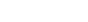The following lemma shows a simple yet useful upper bound for the spectral radius of a matrix:

Lemma: Let A ∈ Cn × n be a complex-valued matrix, ρ(A) its spectral radius and ||·|| a consistent matrix norm; then, for each k ∈ N: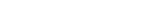'Proof: Let (v, λ) be an eigenvector-eigenvalue pair for a matrix A. By the sub-multiplicative property of the matrix norm, we get: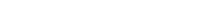and since v ≠ 0 for each λ we have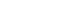and therefore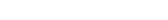The spectral radius is closely related to the behaviour of the convergence of the power sequence of a matrix; namely, the following theorem holds:

Theorem: Let A ∈ Cn × n be a complex-valued matrix and ρ(A) its spectral radius; then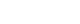if and only if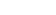Moreover, if ρ(A)>1,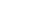is not bounded for increasing k values.

Proof: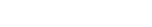Let (v, λ) be an eigenvector-eigenvalue pair for matrix A. Since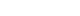we have: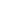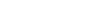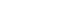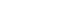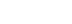and, since by hypothesis v ≠ 0, we must have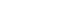which implies |λ| < 1. Since this must be true for any eigenvalue λ, we can conclude ρ(A) < 1.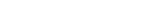From the Jordan normal form
Jordan normal form
In linear algebra, a Jordan normal form of a linear operator on a finite-dimensional vector space is an upper triangular matrix of a particular form called Jordan matrix, representing the operator on some basis...

theorem, we know that for any complex valued matrix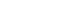, a non-singular matrix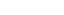and a block-diagonal matrix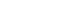exist such that: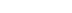withwhereIt is easy to see that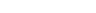and, since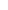is block-diagonal,Now, a standard result on the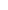-power of an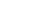Jordan block states that, for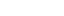:Thus, if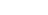then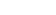, so that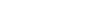which implies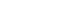Therefore,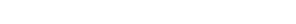On the other side, if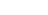, there is at least one element inwhich doesn't remain bounded as k increases, so proving the second part of the statement.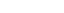## Theorem (Gelfand's formula, 1941)

For any matrix norm ||·||, we have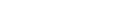In other words, the Gelfand's formula shows how the spectral radius of A gives the asymptotic growth rate of the norm of Ak: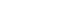for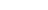Proof: For any ε > 0, consider the matrix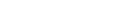Then, obviously,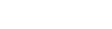and, by the previous theorem,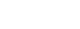That means, by the sequence limit definition, a natural number N1N exists such that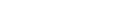which in turn means: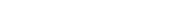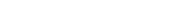Let's now consider the matrix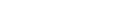Then, obviously,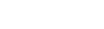and so, by the previous theorem,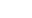is not bounded.

This means a natural number N2N exists such that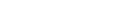which in turn means: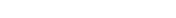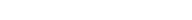Taking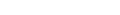and putting it all together, we obtain: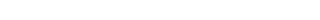which, by definition, is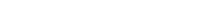Gelfand's formula leads directly to a bound on the spectral radius of a product of finitely many matrices, namely assuming that they all commute we obtain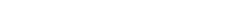Actually, in case the norm is consistent, the proof shows more than the thesis; in fact, using the previous lemma, we can replace in the limit definition the left lower bound with the spectral radius itself and write more precisely: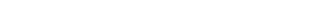which, by definition, is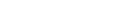Example: Let's consider the matrix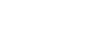whose eigenvalues are 5, 10, 10; by definition, its spectral radius is ρ(A)=10. In the following table, the values of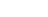for the four most used norms are listed versus several increasing values of k (note that, due to the particular form of this matrix,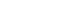):
k
1 14 15.362291496 10.681145748
2 12.649110641 12.328294348 10.595665162
3 11.934831919 11.532450664 10.500980846
4 11.501633169 11.151002986 10.418165779
5 11.216043151 10.921242235 10.351918183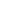10 10.604944422 10.455910430 10.183690042
11 10.548677680 10.413702213 10.166990229
12 10.501921835 10.378620930 10.15303159620 10.298254399 10.225504447 10.091577411
30 10.197860892 10.149776921 10.060958900
40 10.148031640 10.112123681 10.045684426
50 10.118251035 10.089598820 10.036530875100 10.058951752 10.044699508 10.018248786
200 10.029432562 10.022324834 10.009120234
300 10.019612095 10.014877690 10.006079232
400 10.014705469 10.011156194 10.0045590781000 10.005879594 10.004460985 10.001823382
2000 10.002939365 10.002230244 10.000911649
3000 10.001959481 10.001486774 10.00060775710000 10.000587804 10.000446009 10.000182323
20000 10.000293898 10.000223002 10.000091161
30000 10.000195931 10.000148667 10.000060774100000 10.000058779 10.000044600 10.000018232

## Bounded linear operators

For a bounded linear operator A and the operator norm
Operator norm
In mathematics, the operator norm is a means to measure the "size" of certain linear operators. Formally, it is a norm defined on the space of bounded linear operators between two given normed vector spaces.- Introduction and definition :...

||·||, again we haveA bounded operator (on a complex Hilbert space) called a spectraloid operator if its spectral radius coincides with its numerical radius. An example of such an operator is a normal operator
Normal operator
In mathematics, especially functional analysis, a normal operator on a complex Hilbert space H is a continuous linear operatorN:H\to Hthat commutes with its hermitian adjoint N*: N\,N^*=N^*N....

.

## Graphs

The spectral radius of a finite graph
Graph (mathematics)
In mathematics, a graph is an abstract representation of a set of objects where some pairs of the objects are connected by links. The interconnected objects are represented by mathematical abstractions called vertices, and the links that connect some pairs of vertices are called edges...

In mathematics and computer science, an adjacency matrix is a means of representing which vertices of a graph are adjacent to which other vertices...

.

This definition extends to the case of infinite graphs with bounded degrees of vertices (i.e. there exists some real number C such that the degree of every vertex of the graph is smaller than C). In this case, for the graph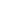let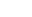denote the space of functions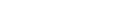with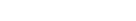. Let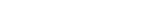be the adjacency operator of, i.e.,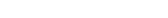. The spectral radius of G is defined to be the spectral radius of the bounded linear operator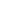.